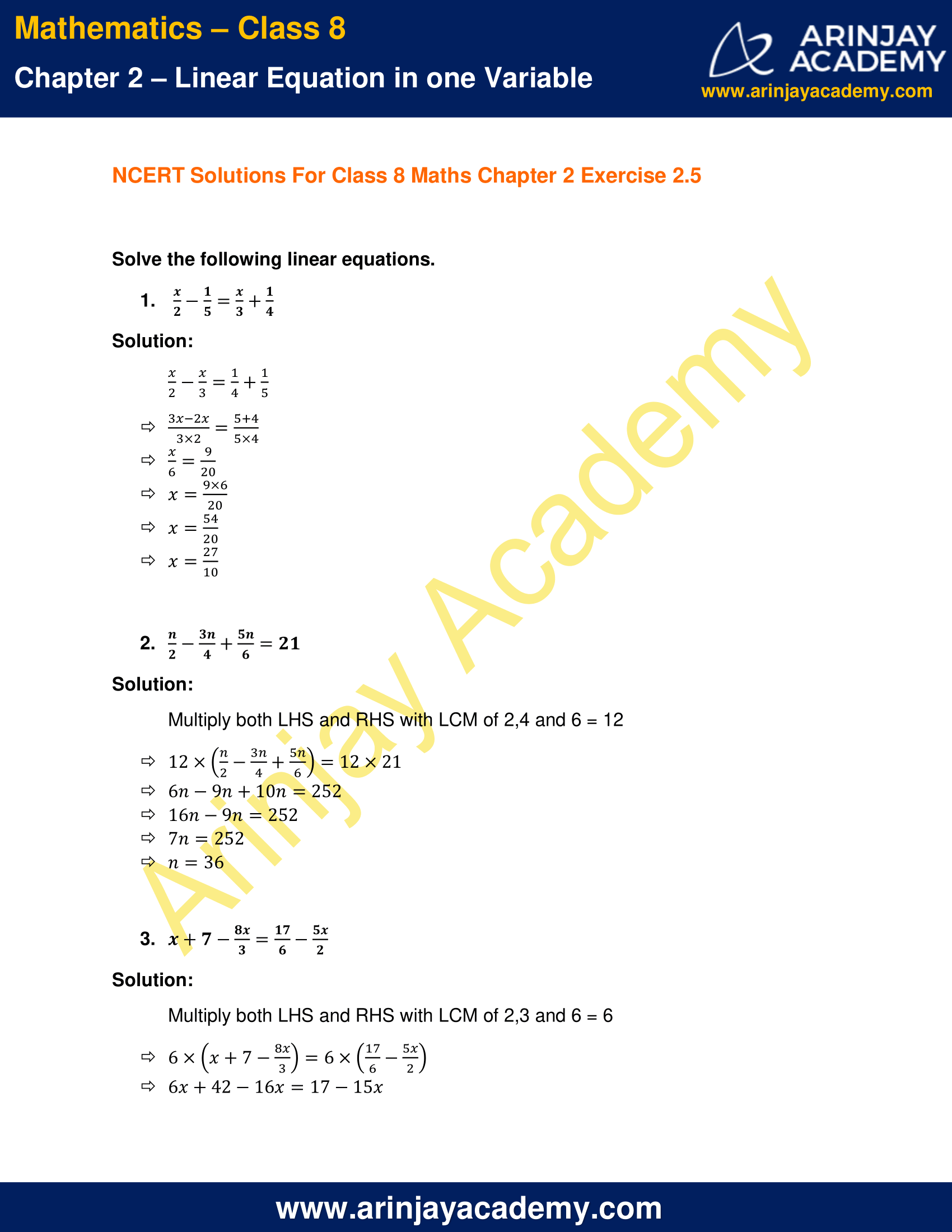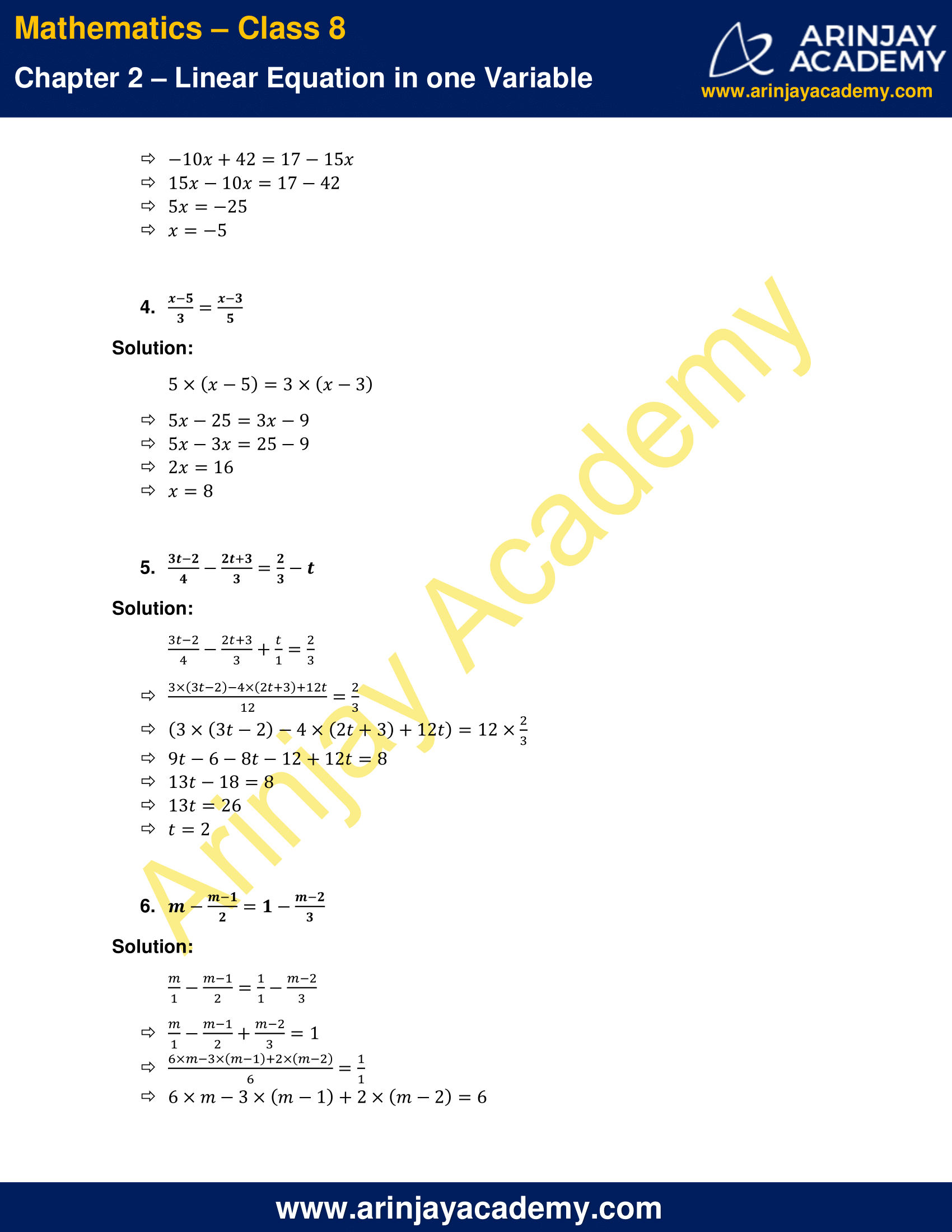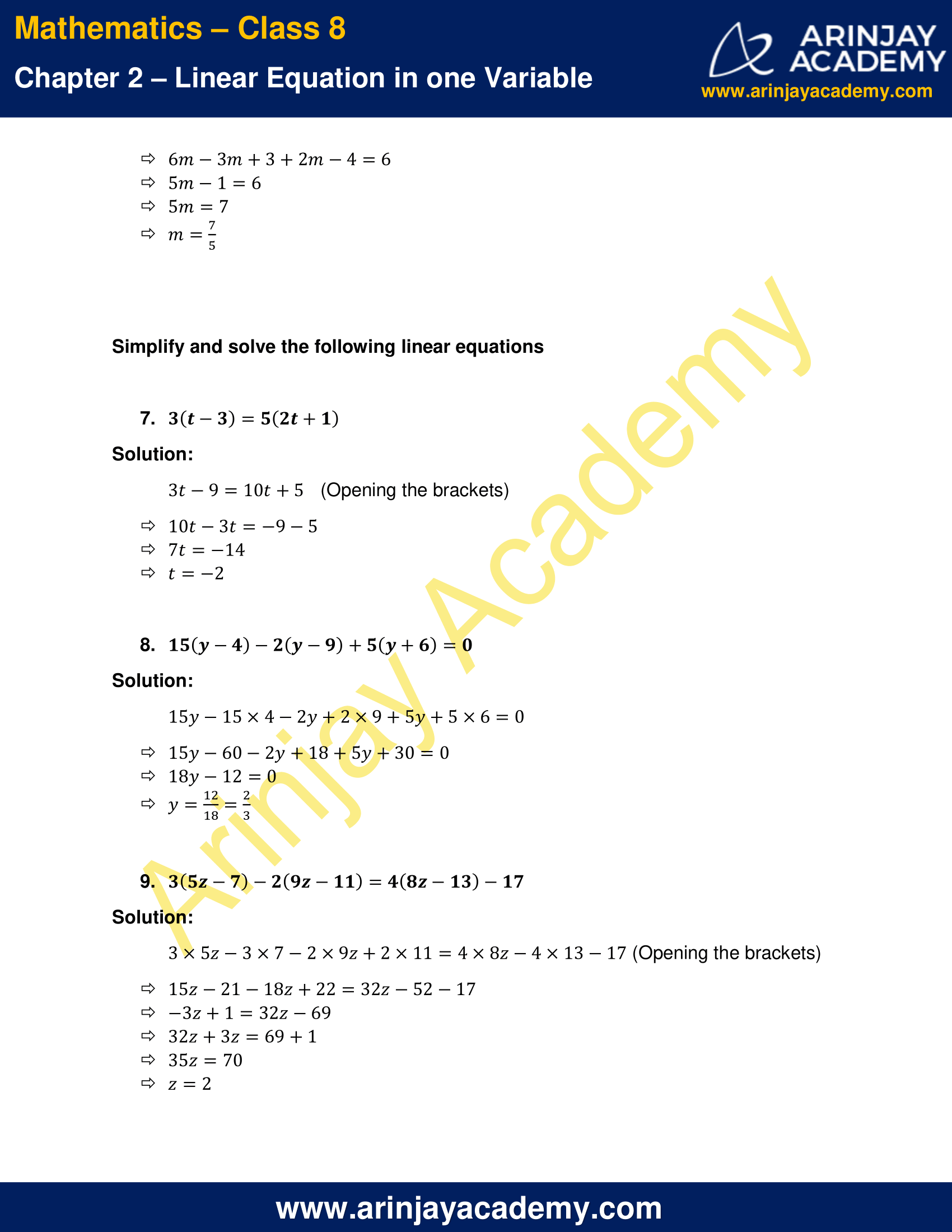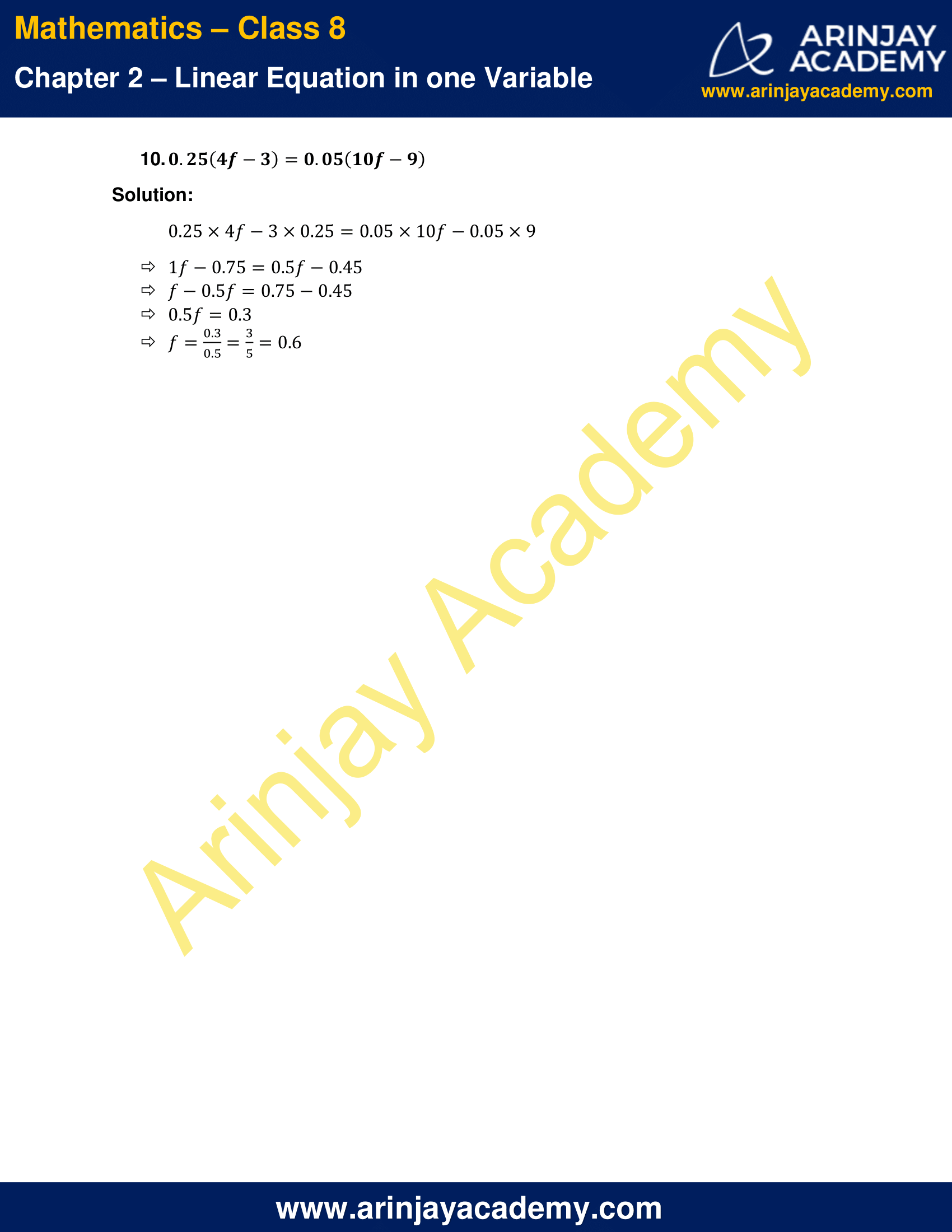# NCERT Solutions for Class 8 Maths Chapter 2 Exercise 2.5

NCERT Solutions for Class 8 Maths Chapter 2 Exercise 2.5 – Linear Equation in one variable, has been designed by the NCERT to test the knowledge of the student on the topic – Reducing Equations to Simpler Form

### NCERT Solutions for Class 8 Maths Chapter 2 Exercise 2.5NCERT Solutions for Class 8 Maths Chapter 2 Exercise 2.5

Solve the following linear equations.

1. (x/2) – (1/5) = (x/3) + (1/4)
Solution:
(x/2)-(x/3) = (1/4) + (1/5)
(3x-2x)/(3×2) = (5+4)/(5×4)
x/6 = 9/20
x = (9×6)/20
x = 54/20
x = 27/10

2. (n/2)-(3n/4)+(5n/6)=21
Solution:
Multiply both LHS and RHS with LCM of 2,4 and 6 = 12
12× [(n/2) – (3n/4) + (5n/6)] = 12×21
6n-9n+10n=252
16n-9n=252
7n=252
n=36

3. x+7 – (8x/3) = (17/6) – (5x/2)
Solution:
Multiply both LHS and RHS with LCM of 2,3 and 6 = 6
6×[x+7- (8x/3)]=6×[(17/6) – (5x/2)]
6x+42-16x=17-15x
-10x+42=17-15x
15x-10x=17-42
5x=-25
x=-5

4. (x-5)/3 = (x-3)/5
Solution:
5×(x-5)=3×(x-3)
5x-25=3x-9
5x-3x=25-9
2x=16
x=8

5. (3t-2)/4 – (2t+3)/3 = (2/3)-t
Solution:
(3t-2)/4 – (2t+3)/3 + (t/1) = 2/3
[3 × (3t-2)-4 × (2t+3)+12t]/12 =2/3
(3×(3t-2)-4×(2t+3)+12t)=12×(2/3)
9t-6-8t-12+12t=8
13t-18=8
13t=26
t=2

6. m – (m-1)/2 = 1 – (m-2)/3
Solution:
(m/1) – (m-1)/2 = (1/1) – (m-2)/3
(m/1) – (m-1)/2 + (m-2)/3 = 1
[6 × m-3 × (m-1)+2 × (m-2)]/6 = (1/1)
6×m-3×(m-1)+2×(m-2)=6
6m-3m+3+2m-4=6
5m-1=6
5m=7
m=7/5

Simplify and solve the following linear equations

7. 3(t-3)=5(2t+1)
Solution:
3t-9=10t+5 (Opening the brackets)
10t-3t=-9-5
7t=-14
t=-2

8. 15(y-4)-2(y-9)+5(y+6)=0
Solution:
15y-15×4-2y+2×9+5y+5×6=0
15y-60-2y+18+5y+30=0
18y-12=0
y = 12/18 = 2/3

9. 3(5z-7)-2(9z-11)=4(8z-13)-17
Solution:
3×5z-3×7-2×9z+2×11=4×8z-4×13-17 (Opening the brackets)
15z-21-18z+22=32z-52-17
-3z+1=32z-69
32z+3z=69+1
35z=70
z=2

10. 0.25(4f-3)=0.05(10f-9)
Solution:
0.25×4f-3×0.25=0.05×10f-0.05×9
1f-0.75=0.5f-0.45
f-0.5f=0.75-0.45
0.5f=0.3
f= 0.3/0.5 = 3/5=0.6

The next Exercise for NCERT Solutions for Class 8 Maths Chapter 2 Exercise 2.6 – Linear Equation in one variable can be accessed by clicking here